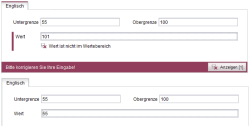## <AND/>: AND operations in dynamic forms

The <AND/> tag can be implemented in the value determination area of the rule definition (or for the definition of a precondition) and is used to link multiple logical expressions. The result of the combined logical expression is a Boolean value, which is true when all linked expressions are true:

`<AND>    Expression 1    Expression 2     ...    Expression n</AND>`

The <AND/> tag is used to check multiple form properties in a rule and to link them with a handling instruction, e.g.:

• “Form page A is displayed if the form was opened in the page store AND the option "Generate this section in the output" was activated.” or
• “If the value of the input component is greater than 0 AND less than 10, a message appears in the form.”

## Examples

### Example: Checking if a number is within a defined value rangeThe following form contains three type CMS_INPUT_NUMBER input components:

“st_lowerLimit” for information on the lower limit of a value range

“st_upperLimit” for information on the upper limit of a value range and

“st_value” for information on a value that is within the specified value range

A dynamic form is used to ensure that the editor enters a numerical value in the input component “st_value” that is within the defined value range. This is ensured when one of the following conditions is fulfilled:

• The value in “st_value” is equal to the value in “st_lowerLimit” OR
• The value in “st_value” is equal to the value in “st_upperLimit” OR
• The value in “st_value” is less than the value in “st_upperLimit” AND greater than the value in “st_lowerLimit”

These conditions are checked within the <WITH/> section of the rule as follows:

1) Is the value from “st_value” equal to the value from “st_lowerLimit”?

`<EQUAL>    <PROPERTY source="st_value" name="VALUE"/>    <PROPERTY source="st_lowerLimit" name="VALUE"/></EQUAL>`

2) Is the value from “st_value” greater than the value from “st_lowerLimit” AND less than the value from “st_upperLimit”?
In this case, both conditions must be met, and therefore they are linked using <AND/>:

`<AND>    <GREATER_THAN>        <PROPERTY source="st_value" name="VALUE"/>        <PROPERTY source="st_lowerLimit" name="VALUE"/>    </GREATER_THAN>    <LESS_THAN>        <PROPERTY source="st_value" name="VALUE"/>        <PROPERTY source="st_upperLimit" name="VALUE"/>    </LESS_THAN></AND>`

3) Is the value from “st_value” equal to the value from “st_upperLimit”?

`<EQUAL>    <PROPERTY source="st_value" name="VALUE"/>    <PROPERTY source="st_upperLimit" name="VALUE"/></EQUAL>`

If one of these three logical expressions is true, the condition (Value from “st_value” must be within the defined value range.) is fulfilled. The expressions can therefore be linked using <OR/>:

`<OR>    <EQUAL>        <PROPERTY source="st_value" name="VALUE"/>        <PROPERTY source="st_lowerLimit" name="VALUE"/>    </EQUAL>    <AND>        <GREATER_THAN>            <PROPERTY source="st_value" name="VALUE"/>            <PROPERTY source="st_lowerLimit" name="VALUE"/>        </GREATER_THAN>        <LESS_THAN>            <PROPERTY source="st_value" name="VALUE"/>            <PROPERTY source="st_upperLimit" name="VALUE"/>        </LESS_THAN>    </AND>    <EQUAL>        <PROPERTY source="st_value" name="VALUE"/>        <PROPERTY source="st_upperLimit" name="VALUE"/>    </EQUAL></OR>`

The result of this combined logical expression is a Boolean value that is then linked in the <DO/> section with the input component “st_value” validation. As long as the condition (“Value is within the defined value range”) is not fulfilled, the <VALIDATION/> section of the rule is executed and a correction notification appears. The SAVE restriction level also prevents saving of the form.

`...<RULE>    <WITH>        <OR>            <EQUAL>                <PROPERTY source="st_value" name="VALUE"/>                <PROPERTY source="st_lowerLimit" name="VALUE"/>            </EQUAL>            <AND>                <GREATER_THAN>                    <PROPERTY source="st_value" name="VALUE"/>                    <PROPERTY source="st_lowerLimit" name="VALUE"/>                </GREATER_THAN>                <LESS_THAN>                    <PROPERTY source="st_value" name="VALUE"/>                    <PROPERTY source="st_upperLimit" name="VALUE"/>                </LESS_THAN>            </AND>            <EQUAL>                <PROPERTY source="st_value" name="VALUE"/>                <PROPERTY source="st_upperLimit" name="VALUE"/>            </EQUAL>        </OR>    </WITH>    <DO>        <VALIDATION scope="SAVE">            <PROPERTY source="st_value" name="VALID"/>            <MESSAGE lang="*" text="Value is not part of codomain"/>            <MESSAGE lang="DE" text="Wert ist nicht im Wertebereich"/>        </VALIDATION>    </DO></RULE>...`